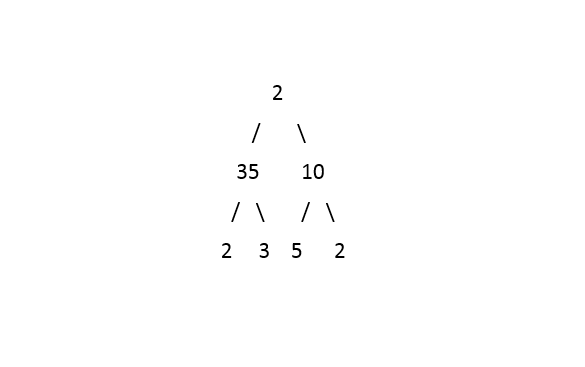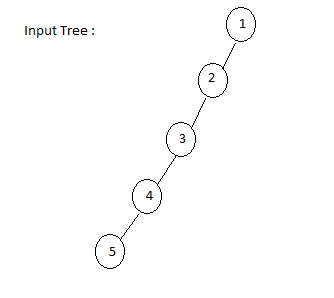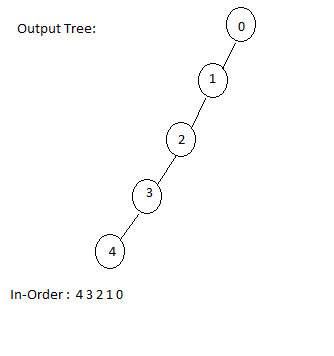Problem title
Difficulty
Avg time to solve

Product Array Puzzle
Easy
15 mins
Easy
15 mins
Constellation
Easy
10 mins
Longest Increasing Path In A 2D Matrix
Hard
10 mins
Check If Linked List Is Palindrome
Easy
15 mins
Data Structure Supporting Insert Delete And GetRandom
Easy
15 mins
Permutation In String
Hard
20 mins
Maximum number in K swaps
Hard
15 mins
Check if the door is open or closed
Easy
10 mins
Minimum Sum Subarray
Easy
15 mins3

# Replace Node With Depth

Difficulty: EASY
Avg. time to solve
20 min
Success Rate
85%

Problem Statement

#### Root is at depth 0, hence the root data is updated with 0. Replicate the same further going down the tree in the depth of the given tree.

##### Input Format:
``````The first line contains an Integer 't' which denotes the number of test cases or queries to be run. Then the test cases follow.

The first line of input contains the elements of the tree in the level order form separated by a single space.
If any node does not have left or right child, take -1 in its place. Refer to the example below.

Example:

Elements are in the level order form. The input consists of values of nodes separated by a single space in a single line. In case a node is null, we take -1 on its place.

For example, the input for the tree depicted in the below image would be :
````````````1
2 3
4 -1 5 6
-1 7 -1 -1 -1 -1
-1 -1

Explanation :
Level 1 :
The root node of the tree is 1

Level 2 :
Left child of 1 = 2
Right child of 1 = 3

Level 3 :
Left child of 2 = 4
Right child of 2 = null (-1)
Left child of 3 = 5
Right child of 3 = 6

Level 4 :
Left child of 4 = null (-1)
Right child of 4 = 7
Left child of 5 = null (-1)
Right child of 5 = null (-1)
Left child of 6 = null (-1)
Right child of 6 = null (-1)

Level 5 :
Left child of 7 = null (-1)
Right child of 7 = null (-1)

The first not-null node (of the previous level) is treated as the parent of the first two nodes of the current level. The second not-null node (of the previous level) is treated as the parent node for the next two nodes of the current level and so on.

The input ends when all nodes at the last level are null (-1).
``````
##### Note :
``````The above format was just to provide clarity on how the input is formed for a given tree.

The sequence will be put together in a single line separated by a single space. Hence, for the above-depicted tree, the input will be given as:

1 2 3 4 -1 5 6 -1 7 -1 -1 -1 -1 -1 -1
``````
##### Output Format :
``````For each test case, print the inorder traversal of the tree where each node is replaced by the depth of the current node.

Output for every test case will be denoted in a separate line.
``````
##### Note :
``````You do not need to print anything, it has already been taken care of. Just implement the given function.
``````
##### Constraints:
``````1 <= T <= 100
1 <= N <= 1000
-10^9 <= data <= 10^9

Time limit: 1 sec
``````
##### Sample Input 1 :
``````1
2 35 10 2 3 5 2 -1 -1 -1 -1 -1 -1 -1 -1
``````
##### Sample Output 1 :
``````2 1 2 0 2 1 2
``````
##### Explanation Of the Sample Input 1 :##### Sample Input 2 :
``````1
1 2 -1 3 -1 4 -1 5 -1 -1 -1
``````
##### Sample Output 2 :
``````4 3 2 1 0
``````
##### Explanation Of the Sample Input 2 :Console